# Introduction

Implementing authentication (i.e. user login) in a system sounds simple. Just compare username and password with what is stored in the database, right?

Actually, it’s not nearly as simple as it looks to the user logging in. Storing and checking user credentials is a process with different levels of security (and I’m no expert, so there may be more):

2. Hash the passwords. They won’t be easily readable, but can be cracked by rainbow tables.
3. Salt and hash the passwords. Protects against rainbow tables as well, but still vulnerable to high-performance brute force attacks.
4. Use a slow hash algorithm to limit the effectiveness of brute force attacks.

My article “Securing Passwords by Salting and Hashing” covers the first three items. This article will deal with the fourth item, and introduce BCrypt, which gives you all four.

# Authentication with BCrypt

Before we discuss the merits of using BCrypt, let’s see how to use it.

You first need to include the BCrypt library in your project. You can do this via NuGet: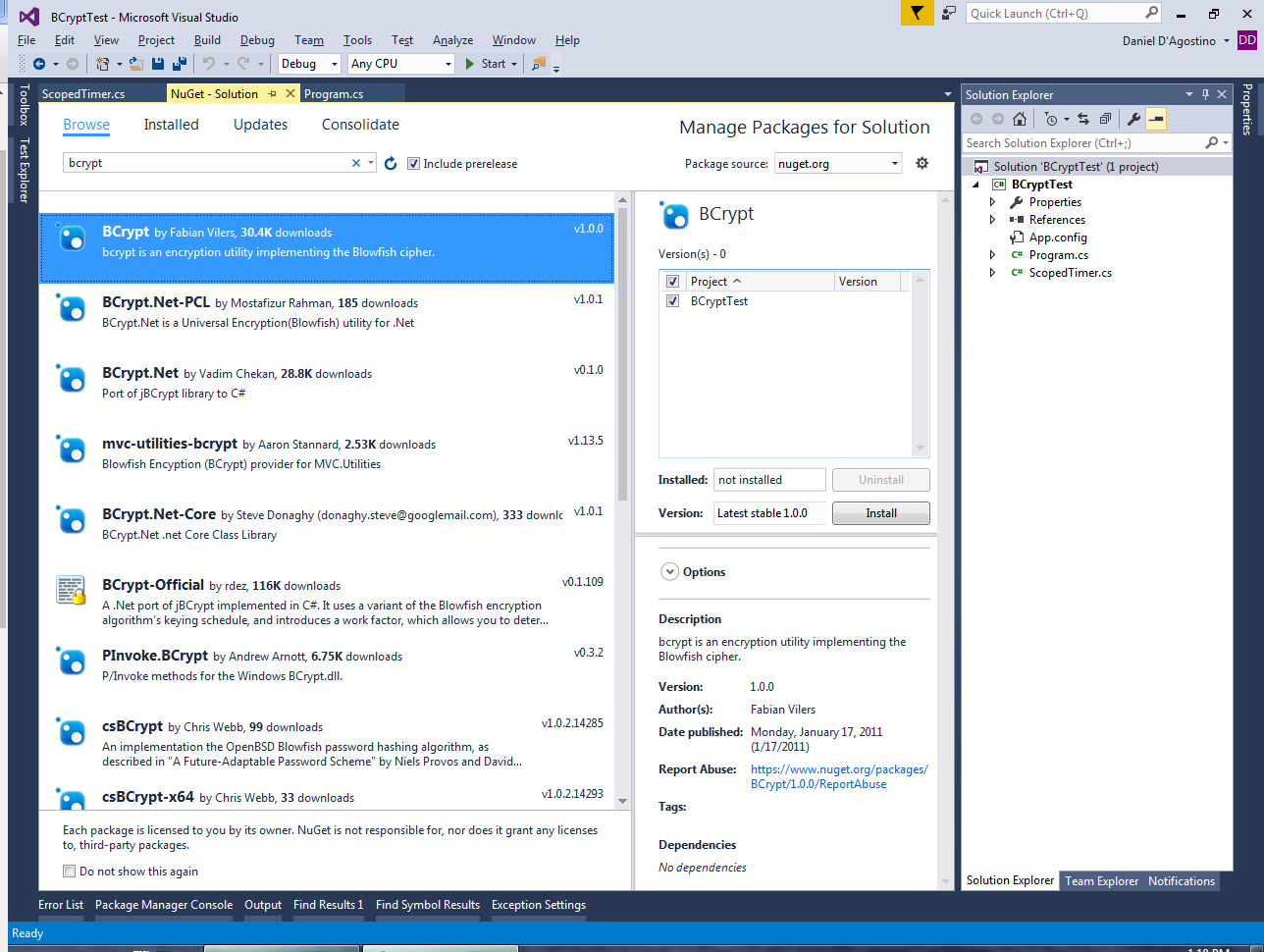The functionality we need to use is all in a class called `BCryptHelper`, which you get access to by including the following namespace:

```using DevOne.Security.Cryptography.BCrypt;
```

With that, it is very easy to generate a salt, hash a password with it, and validate the password against its salted hashed version:

```        static void Main(string[] args)
{
Console.Title = "BCryptTest";

string salt = BCryptHelper.GenerateSalt();

Console.WriteLine("Salt:            {0}", salt);
Console.WriteLine("Valid:           {0}", valid);

}
```

Here’s the output of this little program: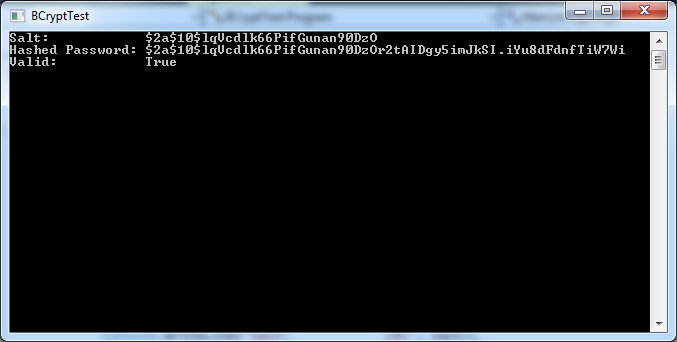The BCrypt hashed password is typically a 60-byte string. As you can see, the salt is actually embedded within the hashed password (this StackOverflow answer explains more about how this works). This means you don’t need to store the salt separately.

# Why BCrypt?

The functionality we have seen in the previous section doesn’t really give us anything more than hashing and salting with any other reasonably strong hash function. So why use BCrypt?

In many programming situations, writing code that executes fast is a good thing. Authentication is not one of those. If the algorithms you use to authenticate your users are fast, that means that brute force attacks may attempt large amounts of combinations per second – more so with modern hardware and GPUs.

Algorithms such as PBKDF2 and BCrypt differ from traditional hash algorithms such as MD5 or SHA256 in that they take a work factor as an input. That is, you can decide how fast or slow the algorithm runs. So if, for instance, you set up your algorithm to take 1 second to validate a password, that greatly limits the possibilities of brute force attacks when compared to algorithms that can run several hundreds or thousands of times per second. Read more about why BCrypt is badass at this Security StackExchange answer.

In BCrypt, the `GenerateSalt()` method takes an optional `logRounds` parameter that affects the performance of subsequent hash operations. It has a default value of 10 and can be set to a number between 4 and 31. The algorithm will run 2 to the power of `logRounds` times, making it run exponentially slower. To get an idea of this, I wrote some simple benchmarking code with the help of my trusted ScopedTimer class (from “Scope Bound Resource Management in C#“):

```        static void GenerateSaltBenchmarks(string password)
{
for (int i = 10; i < 16; i++)
{
using (var scopedTimer = new ScopedTimer(\$"GenerateSalt({i})"))
{
string salt = BCryptHelper.GenerateSalt(i);
}
}
}
```

Here are the results: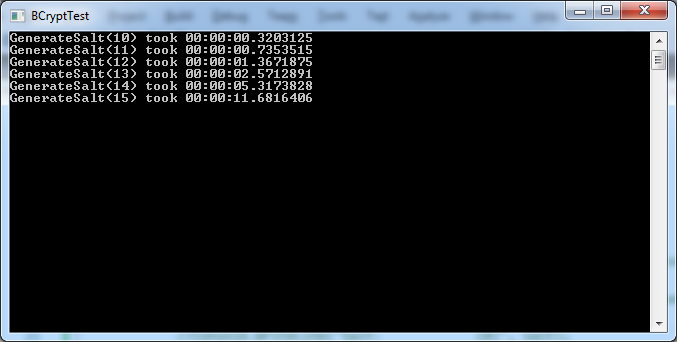# Summary

Use BCrypt to securely store and validate your passwords. It’s easy to use, easy to store, and hard to break. Also importantly, you can make it as slow as you like.

# Data-Driven Tests with NUnit

This article shows how you can run the same unit test multiple times with different input data, using NUnit.

# A Simple Scenario

Before we can learn about data-driven testing using NUnit, we need some actual logic to test. Let’s use the following class for this purpose:

```    public class PriceCalculator
{

{
}

public decimal CalculatePrice(int age)
{
decimal multiplier = 0.0m;

if (age >= 5 && age < 16) // 5 to 15: half price
multiplier = 0.5m;
else if (age >= 16 && age < 60) // 16 to 59: full price
multiplier = 1.0m;
else if (age >= 60) // 60+: half price
multiplier = 0.5m;

}
}
```

So, here we have a scenario where we have something to sell (whatever it is), and certain age ranges get discounts. Kids under 5 years of age are free; older kids under 16 pay half price, as do senior citizens from age 60 onwards.

If you want to run NUnit unit tests from within Visual Studio, make sure you have the NUnit Test Adapter. You can get it from Tools menu -> Extensions and Updates…: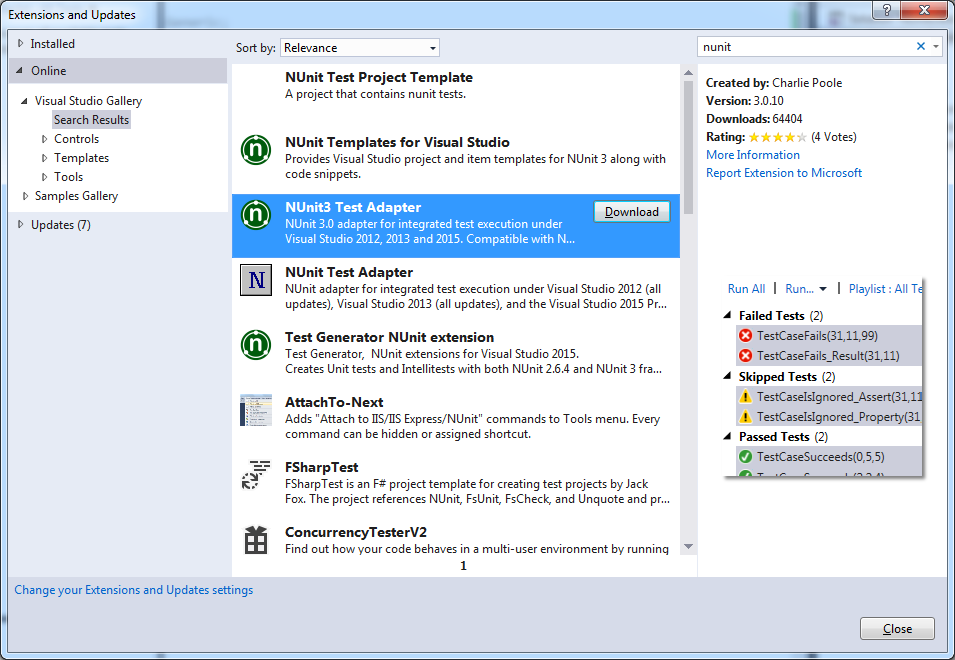# Plain Old Unit Tests

To test this, you’d have to write several unit tests. One of them could look like this:

```        [Test]
public void CalculatePriceTest()
{
// arrange

const int age = 10;

// act

decimal actualPrice = priceCalculator.CalculatePrice(age);

// assert

const decimal expectedPrice = 5m;

Assert.AreEqual(expectedPrice, actualPrice);
}
```

You’d have to write other similar tests to cover the various age ranges and edge cases (e.g. making sure that age boundaries are inclusive or exclusive as needed).

Copying this test code and varying the input data is a bit of a hassle and not quite DRY. An alternative could be to set up a list with the input data and expected result and iterate over it. That’s not necessary, because NUnit gives us a way to do this out of the box.

# The TestCase Attribute

NUnit provides a feature called Parameterized Tests. These provide two mechanisms that allow you to easily run the same test with multiple input data.

The first of these is the [TestCase] attribute. This replaces the [Test] attribute we used before, and provides input data to pass to the test method via parameters:

```        [TestCase(1, 0)]
[TestCase(10, 5)]
[TestCase(20, 10)]
[TestCase(70, 5)]
public void CalculatePriceTest(int age, decimal expectedPrice)
{
// arrange

// act

decimal actualPrice = priceCalculator.CalculatePrice(age);

// assert

Assert.AreEqual(expectedPrice, actualPrice);
}
```

This can actually be simplified further by using the `ExpectedResult` named parameter, and replacing the `Assert` with a simple `return` statement:

```        [TestCase(1, ExpectedResult = 0)]
[TestCase(10, ExpectedResult = 5)]
[TestCase(20, ExpectedResult = 10)]
[TestCase(70, ExpectedResult = 5)]
public decimal CalculatePriceTest(int age)
{
// arrange

// act

decimal actualPrice = priceCalculator.CalculatePrice(age);

// assert

return actualPrice;
}
```

Note: technically we should declare the expected results as `decimal`s in this case, such as `ExpectedResult = 0m`. However, the attribute doesn’t seem to allow it. Integers work just fine.

# The TestCaseSource Attribute

The [TestCase] attribute works great when you want to run the same test against a few different combinations of input data and expected results. However, it can still get very tedious for very exhaustive tests with a lot of input data.

A more fitting technique for such scenarios is to use the [TestCaseSource] attribute:

```        [TestCaseSource("TestCases")]
public decimal CalculatePriceTest(int age)
{
// arrange

// act

decimal actualPrice = priceCalculator.CalculatePrice(age);

// assert

return actualPrice;
}
```

The [TestCaseSource] attribute takes the name of a public static property which will provide the input data (i.e. the same data that we passed in to the [TestCase] attribute earlier). You can use this to load the data from arbitrary sources such as databases, files, etc.

There are a number of ways of working with the [TestCaseSource] attribute (refer to the documentation), but a straightforward way to work with it is to use the `TestCaseData` class. You can provide it with any input data as well as an expected result. For instance, say we have the following CSV file mapping age to expected price, for ages between 0 and 99:

```0,0
1,0
2,0
3,0
4,0
5,5
6,5
7,5
8,5
9,5
10,5
11,5
12,5
13,5
14,5
15,5
16,10
17,10
18,10
...
```

We can write a property that reads this and provides the necessary test case data as follows:

```        public static List<TestCaseData> TestCases
{
get
{
var testCases = new List<TestCaseData>();

using (var sr = new StreamReader(fs))
{
string line = string.Empty;
while (line != null)
{
if (line != null)
{
string[] split = line.Split(new char[] { ',' },
StringSplitOptions.None);

int age = Convert.ToInt32(split);
decimal expectedPrice = Convert.ToDecimal(split);

var testCase = new TestCaseData(age).Returns(expectedPrice);
}
}
}

return testCases;
}
}
```

You can see the power of this approach when you run a hundred tests in the bat of an eyelid: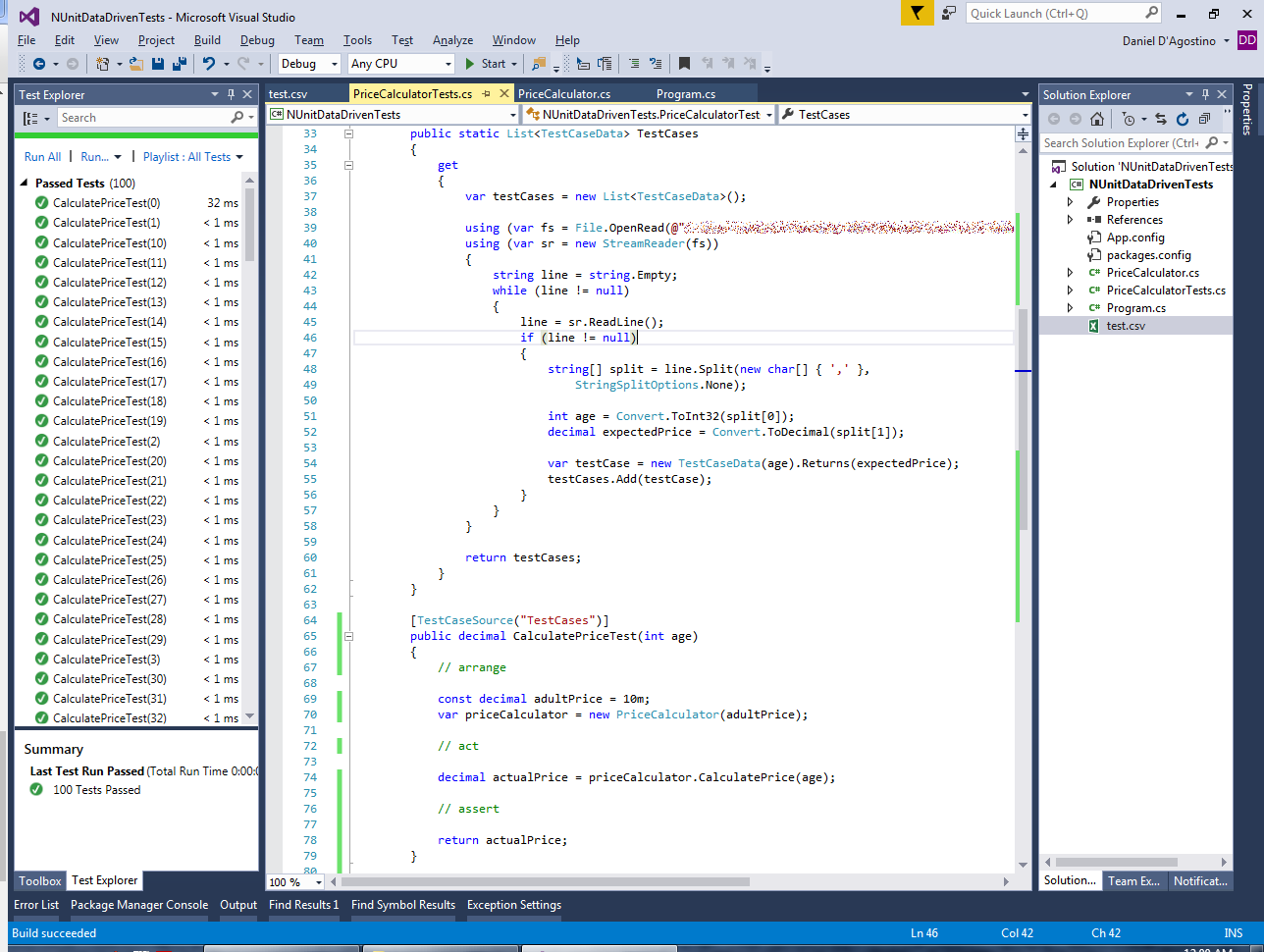The first test takes a small performance penalty as the test data is loaded. But that’s a small price to pay for the development time saved in writing unit tests.